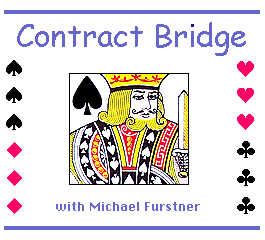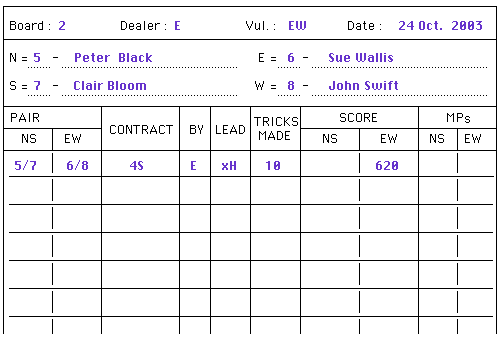Main page - Lessons - Score Caculator Contract Bridge MATCH POINT SCORING

(
Down - Up - Top)

SC 2.1 - The Number of Match Points per Table

Results are scored by allocating match points to each score for each board.
The more pairs compete for each board the higher the match points to be divided.
The lowest point score receive nil points, the second lowest 2 points, the score above that 4 points, and so on.

A set number of match points per board are divided between the NS and EW pair on each table.
The total match points per board to be divided on each table depends on the number of pairs playing that board.

Number of
Tables
Match Points
per Table
2
3
4

5
6
7

8
10
etc.

2
4
6

8
10
12

14
16
etc.

Above listing assumes that each board is played on all tables.
If at the end of the play session some boards were not played on all tables then the match points for that board are also less.

For example if a board was only played on 3 of the 4 tables in play then only 4 match points (MPs) per table are allocated for that board.

(
Down - Up - Top)

SC 2.2 - A Simple Match Point score

EXAMPLE 1 = 4 Tables : 6 match points per board
6 points are divided amongst the 2 pairs on each table.

BOARD 1
Contract by Tricks made NS score EW score NS MPs EW MPs
3 NT NS pair 1 9 +400 (-400) 4 2
3 NT NS pair 2 10 +430 (-430) 6 0
2 NT NS pair 3 10 +150 (-150) 2 4
3 NT NS pair 4 8 (-50) +50 0 6

Be aware that a positive score for one side is at the same time a negative score for the opposition.
A score of 400 for NS is a score of minus 400 for EW. The negative scores are not recorded on the score sheet.

(
Down - Up - Top)

SC 2.3 - Averaging Match points

When 2 or more tables have the same score their combined match points are averaged and equally divided amongst the tied pairs.
For example on Board 2 below :
EW pair 2 and EW pair 3 have a shared 'top'. They share equally their combined match points of 6 + 4 = 10 points, and each pair receives 5 match points.

BOARD 2
Contract by Tricks made NS score EW score NS MPs EW MPs
2 Spades EW pair 1 8 - 110 6 0
2 Spades EW pair 2 9 - 140 1 5
2 Spades EW pair 3 9 - 140 1 5
1 NT EW pair 4 8 - 120 4 2

On Board 3 below an example where three pairs with the same point score equally share the match points.

BOARD 3
Contract by Tricks made NS score EW score NS MPs EW MPs
1 NT EW pair 1 6 50 - 6 0
2 Clubs EW pair 2 8 - 90 2 4
2 Clubs EW pair 3 8 - 90 2 4
2 Clubs EW pair 2 8 - 90 2 4

When a hand is Passed in (no bid other than "Pass" was made) each side scores nil trick- and bonus- points, but still share in the Match points as shown in Board 4 below.

BOARD 4
Contract by Tricks made NS score EW score NS MPs EW MPs
1 NT NS pair 1 7 90 - 6 0
Passed In NS & EW pairs 2 - 0 0 4 2
1 NT NS pair 3 6 - 50 1 5
1 NT NS pair 4 6 - 50 1 5

(
Down - Up - Top)

SC 2.4 - Match pointing an 'Average score'

When on a table the play of a Board is not completed within the allocated time (in a tournament) or some irregularity occurred which made play of the board invalid the Director of the competition may assign to that table an Average score.

The procedure in such a case is as follows :

1. Both NS and EW on the table in question receive an 'Average score', which is half the number of match points of the normal total.
(For 4 tables half of 6 MPs = 3 MPs)

2. The other tables are Match pointed assuming their was one table less in play.
(4 - 1 = 3 tables ---> 4 MPs per table)

3. in addition all pairs on the unaffected tables are given 1 extra MP.

An example is shown on Board 5 below, where the pairs NS4 and EW4 have received an 'Average score'.

BOARD 5
Contract by Tricks made NS score EW score NS MPs EW MPs
3 NT NS pair 1 9 400 - 2 + 1 = 3 2 + 1 = 3
4 Spades NS pair 2 10 420 4 + 1 = 5 0 + 1 = 1
4 Spades NS pair 3 9 - 50 0 + 1 = 1 4 + 1 = 5
Ave. NS & EW pair 4 - - - 3 3

(
Down - Up - Top)

SC 2.5 - Calculating Percentages

The percentage score is the actual match point total a pair wins divided by the maximum total theoretical possible (= 100%).

For example for a 4 Table movement playing 18 boards the theoretical maximum score is :

6 (MPs per table) x 18 (boards) = 108 MPs =100%

For example assume that the NS players had the following match point totals during a play session.
NS1 = 58 points ----> percentage = 58/108 = 53.70 %

NS2 = 64 points ----> percentage = 64/108 = 59.26 %

NS3 = 40 points ----> percentage = 40/108 = 37.04 %

NS4 = 54 points ----> percentage = 54/108 = 50.00 %

Percentages above 50% are above averagescores
Percentages below 50% are below average scores

The total number of MPs distributed over a session is :

MPs per table   x   Number of Boards played   x   Number of Tables (= 4 x 100%)

Each side receives half of this (2 x 100%). Therefore the MP total of all NS pairs (likewise for all EW pairs) should be 216 MPs.
Above example adds up correctly to that amount. This is always used to check that all MPs were calculated and added up correctly.

(Down - Up - Top)

SC 2.6 - Calculating Handicaps

Pairs or individual players can be given a handicap according to their average percentage score over a period of time, by bringing their average up or down to 50%.

Example 1
A player who received these percentage scores over 5 weeks :

49.05% + 44.77% + 51.02% + 46.45% + 40.82% = total 232.11%

has an average score of 46.42%
His handicap is therefore 50.00 - 46.42 = + 3.58

His/her handicap result = actual percentage score + 3.58 (handicap)

If his next result is 48.40% then his handicapped result will be 48.40 + 3.58 = 51.98%

Example 2
A player who received these percentage scores over 5 weeks :

55.04% + 51.63% + 47.97% + 59.32% + 57.48% = total 271.44%

has an average score of 54.29%
His handicap is therefore 50.00 - 54.29 = - 4.29

His handicap result = actual percentage score - 4.29 (handicap)

If his next result is 53.70% then his handicapped result will be 53.70 - 4.29 = 49.41%

A player can be given a handicap after playing at least 5 times in a club.
The handicap is usually recalculated after 10 weeks, leaving out the worst and the best result and averaging the remaining 8 scores.

Handicaps should be reviewed regularly, always taking the last 10 results.

It is best to give each player an individual handicap (even if playing consistently with the same partner).
This handicap can be used when playing occasionally with someone else.

Handicap for a pair should be the average handicap between the two.

Player 1 = -3.58       Player 2 = + 4.29       Pair handicap = +0.35

(
Up - Top)

SC 2.7 - Score sheet Example

Here is a sample traveling Score sheet (called 'traveler') on which all scores and Match Point allocations can be calculated.

On the example below the players compete in an individual movement where each player has his own number and plays during the course of the session with several different partners.Depending on the resolution setting of your computer monitor you may need to enlarge or reduce the printout of the 'traveler'.

(Up - Top)